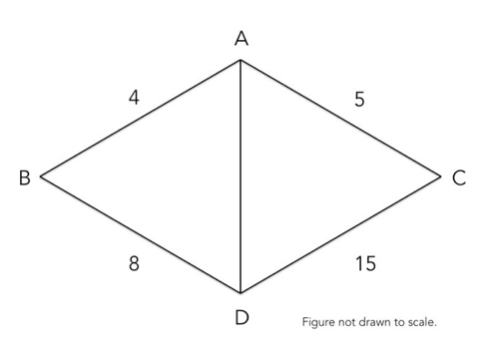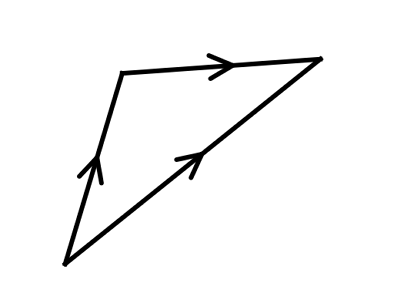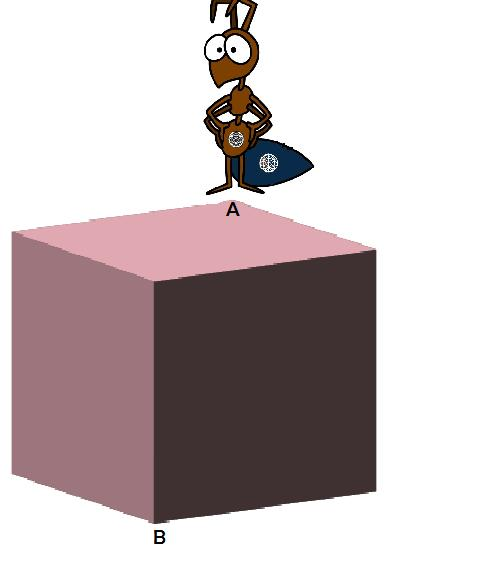Algebra

# Geometric Inequalities: Level 3 Challenges

For all rectangles with perimeter $P$ and area $A$, what is the minimum value of $\frac{P^2}{A}?$If $AB = 4$, $AC = 5$, $BD = 8$, $CD = 15$, and $AD$ is a positive integer, find $AD$.Over all real numbers $x$, find the minimum value of $\sqrt{(x+6)^2+25} + \sqrt{(x-6)^2+121}$.Brilli the ant is trapped on a cube-shaped planet. She wants to go from one corner [point$A$] to the opposite corner [point $B$]. However she wants do this in a way such that she has to cross the shortest distance possible. If the length of the sides of the cube is $1$, the shortest distance between $A$ and $B$ can be expressed as $p+\sqrt{q}$ where $p$ and $q$ are non-negative integers and $q$ is square-free. What is $q-p$?

Details and assumptions:

Brilli the ant is completely confined to the surface of the cube. She can't move inside or outside the cube.A triangle with integral sides has two sides equal to 1 unit. What is the measure of the angle included between the two sides?